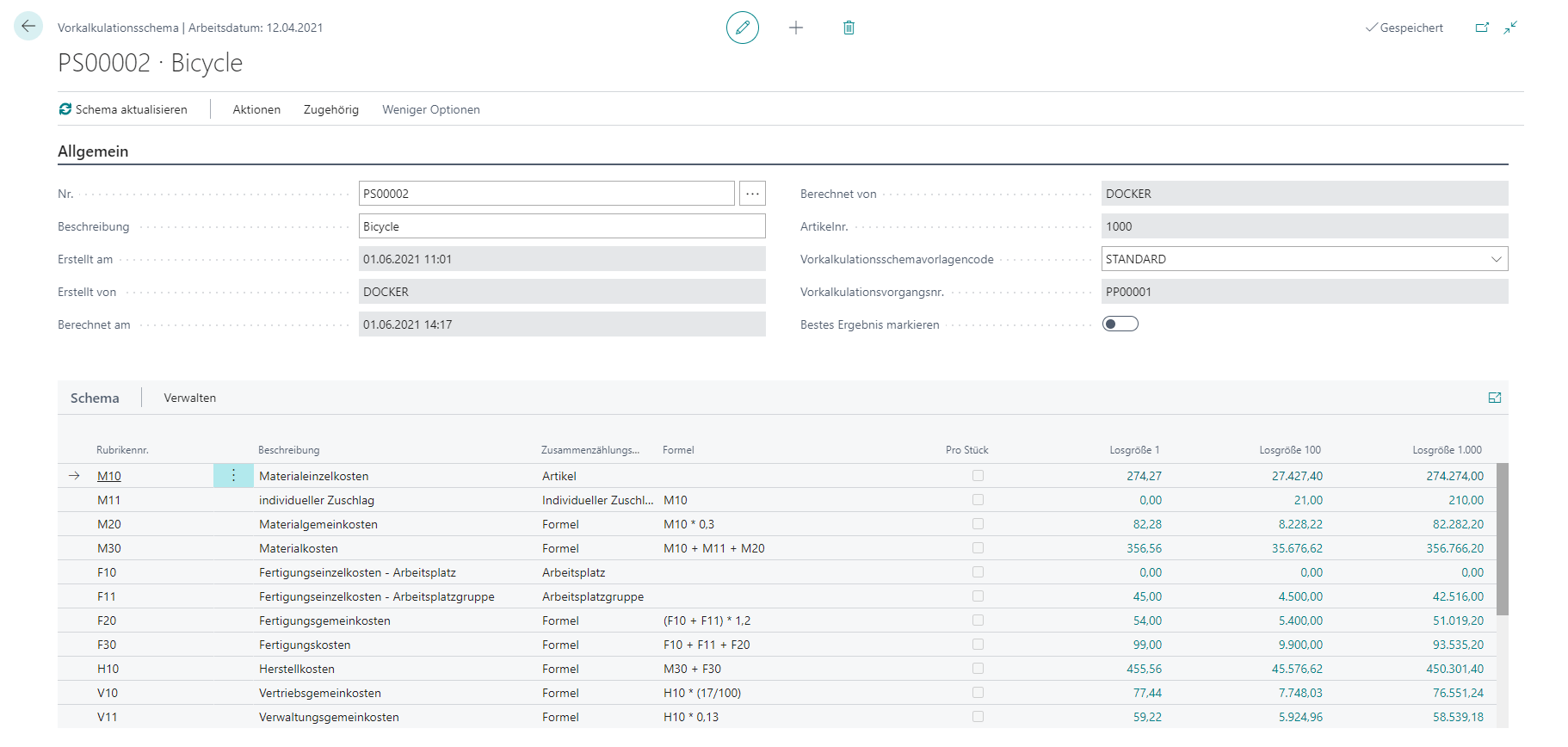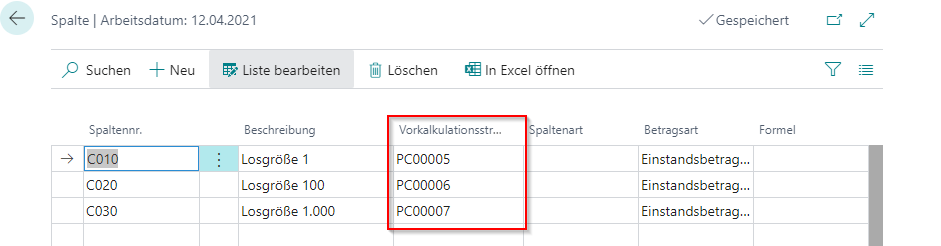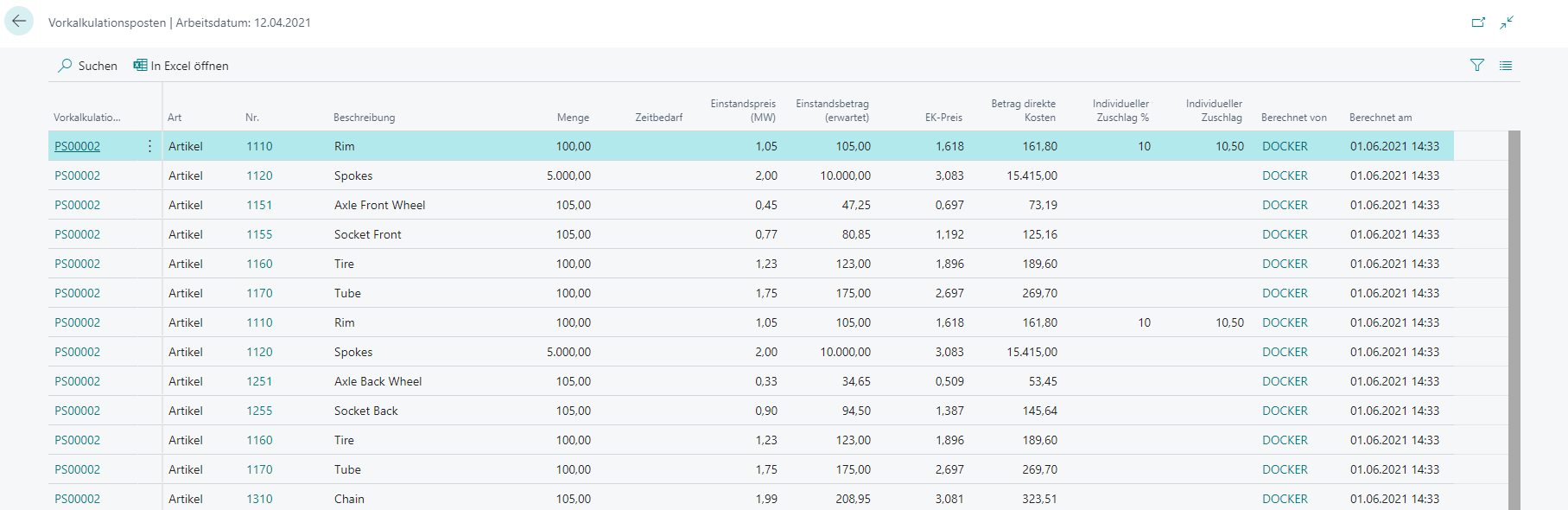# SchemasThe precalculation schemes are for the evaluation of the individual processes.
If you do not have a precalculation schema template stored, you can also enter the schemas manually.The row layout and column layout is similar to the logic in the schema templates. The only difference is that the columns must be linked to a calculation. Thus, only a connection between a schema and the associated calculation can be established.In the case of a template, this link is created automatically.
If you change anything in the schema, you need to update the schema. After that, the corresponding entries will be recalculated.

Mark best result

If the “Mark best result” field is active, the lowest result is displayed. The goal is to find the lowest “Per piece” price.
The lowest “by piece” price in the scheme is decisive. If this is found, the last cell (combination of last row and found column) is marked green.

### Calculation Entries and Calculation Values

Calculation Entries

When updating the schema, so-called “calculation entries” are created. These form the basis for further evaluation.For items, work centers and machine centers the individual lines of the calculation are transferred. At this point, the filters of the schema are also taken into account. If, for example, only a certain item category is evaluated, only the corresponding articles appear in the items for this line of the schema. The individual surcharges are also calculated here, in case they are required.

You can open the entries via the lookup, in which you click on the number of a row with matching totlaing type.

Calculation Values

The calculation values are the “results” of the calculation. For each combination of row (line) and column, the associated value is logged there and displayed in the mask.